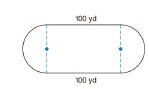Chapter 8.4, Problem 36EElementary Geometry For College St...

7th Edition
Alexander + 2 others
ISBN: 9781337614085

Solutions

Chapter
SectionElementary Geometry For College St...

7th Edition
Alexander + 2 others
ISBN: 9781337614085
Textbook Problem

In exercises 34-45, use your calculator value of π unless otherwise stated. Round answers to two decimal places.A track is to be constructed around the football field at a high school. If the straightaways are 100 yd in length, what length of radius is needed for each of the semicircle shown if the total length around the track is to be 440 yd.To determine

To Find:

The length of the radius of the semi-circular pathway of the football field.

Explanation

Calculation:

A total length around the track is 440 yd.

The length of the straight ways are 100+100=200.

Therefore, the length of the straigntaways is 200yd.

The length of the semi-circular pathways is 440200=220.

Therefore, the circumference of the circular pathway is 220 yd.

We know that the circumference of the circle is C=2πr

Still sussing out bartleby?

Check out a sample textbook solution.

See a sample solution

The Solution to Your Study Problems

Bartleby provides explanations to thousands of textbook problems written by our experts, many with advanced degrees!

Get Started

Evaluate the indefinite integral. x3x2+1dx

Single Variable Calculus: Early Transcendentals, Volume I

In problems 13-16, Use notation to indicate which set is a subset of the other. 14.

Mathematical Applications for the Management, Life, and Social Sciences

In Exercises 1926, pivot the system about the circled element. [1268|32]

Finite Mathematics for the Managerial, Life, and Social Sciences

The graph of is: a) b) c) d)

Study Guide for Stewart's Multivariable Calculus, 8th